# Experiment_609_Determining the Molar Mass Using Ideal Gas Law_1_2_0

$$\newcommand{\vecs}{\overset { \rightharpoonup} {\mathbf{#1}} }$$ $$\newcommand{\vecd}{\overset{-\!-\!\rightharpoonup}{\vphantom{a}\smash {#1}}}$$$$\newcommand{\id}{\mathrm{id}}$$ $$\newcommand{\Span}{\mathrm{span}}$$ $$\newcommand{\kernel}{\mathrm{null}\,}$$ $$\newcommand{\range}{\mathrm{range}\,}$$ $$\newcommand{\RealPart}{\mathrm{Re}}$$ $$\newcommand{\ImaginaryPart}{\mathrm{Im}}$$ $$\newcommand{\Argument}{\mathrm{Arg}}$$ $$\newcommand{\norm}{\| #1 \|}$$ $$\newcommand{\inner}{\langle #1, #2 \rangle}$$ $$\newcommand{\Span}{\mathrm{span}}$$ $$\newcommand{\id}{\mathrm{id}}$$ $$\newcommand{\Span}{\mathrm{span}}$$ $$\newcommand{\kernel}{\mathrm{null}\,}$$ $$\newcommand{\range}{\mathrm{range}\,}$$ $$\newcommand{\RealPart}{\mathrm{Re}}$$ $$\newcommand{\ImaginaryPart}{\mathrm{Im}}$$ $$\newcommand{\Argument}{\mathrm{Arg}}$$ $$\newcommand{\norm}{\| #1 \|}$$ $$\newcommand{\inner}{\langle #1, #2 \rangle}$$ $$\newcommand{\Span}{\mathrm{span}}$$$$\newcommand{\AA}{\unicode[.8,0]{x212B}}$$

 Student Name Laboratory Date:  Date Report Submitted: ___________________________ Student ID Experiment Number and Title Experiment 609:  Molar Mass Determination Using Ideal Gas Law

Experiment 609:  Molar Mass Determination Using Ideal Gas Law

Section 1:  Purpose and Summary

Determine the molar mass of an unknown volatile liquid by measuring the properties of its vapor phase after heating.  Using the properties of its vapor phase, calculate its molar mass using the ideal gas law.

In this experiment, students will determine the molar mass of an unknown volatile liquid by measuring the properties of its vapor phase after heating. The liquid is heated until it is completely vaporized. The vapor phase is trapped in a flask and the properties of the vapor are measured. The molar mass of the sample is then calculated using the ideal gas law:

PV = nRT

where P is the pressure in atmospheres, V is the volume in liters, n is the number of moles of the gas, R is the gas constant, 0.0821 $$\frac{\text {L× atm}}{\text {mol× K}}=$$ , and T is the Kelvin temperature.

Section 2:  Safety Precautions and Waste Disposal

Safety Precautions:

Use of eye protection is recommended for all experimental procedures.

Use tongs or mitts to handle hot flasks and beakers.

Waste Disposal:

Discard any excess unknown liquids in the organic waste container in the fume hood.

Section 3: Procedure

Liquid sample in this experiment may be any of the following:  acetone, isopropyl alcohol, ethyl acetate, or cyclopentane.  Additional liquids may also be added at the request of the instructor.

 1.  Obtain a liquid sample from your instructor.  Your instructor may choose to issue the liquid as an unknown sample. Record the name of the liquid sample here, or the identification number of the unknown:

 2. Obtain a clean, dry 125-mL Erlenmeyer flask, add one small crystal of dithizone (a dye) about the size of a grain of salt,  and loosely cover it with a square piece of aluminum foil (about 5 cm x 5 cm). Secure the aluminum foil by tying a copper wire.  It should look something like the following: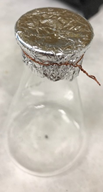The crystal of dithizone is in the photo. It is very small.  You do not need much dye.  Weigh the flask, cover, dithizone crystal, and copper wire. Record mass to at least the nearest 1 milligram (0.001 grams). Mass of Erlenmeyer flask with cover, dithizone and copper wire    ______________________ grams 3. Carefully remove the copper wire and aluminum foil cover and transfer about 5 mL of the liquid sample into the flask. It is important to not lose any of the foil or copper wire. 4. Replace foil cover and crimp it tightly around the opening of the flask. Secure the foil with the copper wire. Make sure that there is no loose foil hanging down that may collect moisture from the water bath during the experiment. 5. Use a pin to poke 1 small pinhole on the foil cover of the flask to allow the air and excess vapor to escape and maintain a pressure inside the flask that is equal to the atmospheric pressure. 6.  Prepare a water bath by filling a 600-mL beaker with about 400 mL of tap water. Add a few boiling chips. Place it on a wire gauze on an iron ring attached to a ring stand. Adjust the height of the ring to make sure that the hottest part of the flame of the Bunsen burner touches the bottom of the beaker. The beaker can be secured with an oversize ring to prevent it from falling over. 7. Carefully attach a clamp to the neck of the flask and lower the flask, slightly tilted, as deep into the hot water bath as possible. Secure the clamp to the ring stand.  One way to set up the apparatus is shown in the picture on the left.    The thermometer is held in place with a buret clamp or a thermometer clamp.      Other thermometers may be used as needed or available.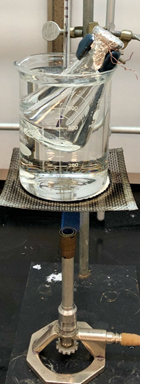8. Begin heating the water bath. Occasionally stir the water in the water bath with a stirring rod. Monitor the temperature of the water bath with a thermometer. Maintain the temperature of the water at around 95°C, or just below boiling throughout this experiment.  Add additional water as needed to maintain the level of water.    The wire screen is necessary to disperse the heat from the flame to avoid breaking the beaker due to thermal shock.    Alternatively, a different setup may be used with hot plates instead of Bunsen burners. Check with your instructor as to the specific procedure to use.    Use enough water to submerge a 125-ml Erlenmeyer flask but not enough to overflow the beaker.  The thermometer is not shown.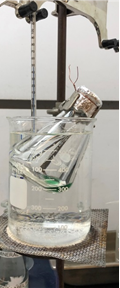9.  Continue heating and watch the green color of the dithizone disappear – this indicates that the liquid sample has completely vaporized. If the water starts to boil while the liquid is evaporating, record this observation.     The dithizone dye is intensely green in solution, but dries to a dark solid residue.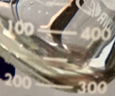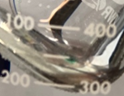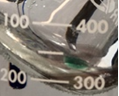10. When you are sure that the liquid has completely vaporized, record the temperature of the water bath. Turn off the Bunsen burner.  The pictures below show the close up of what the evaporating materials look like.      Liquid present Almost gone      Liquid gone Temperature of the water bath    Trial 1: __________________ °C    Trial 2: __________________ °C 11. Carefully remove the flask from the water bath by raising the clamp on the ring stand. The clamp may be hot; use thermal gloves or paper towels to protect from heat, if necessary.   Keep the flask secured in the clamp until it has cooled to room temperature. Cooling may also be done faster by submerging the flask in an ice bath. While waiting for the flask to cool to room temperature, obtain the barometric pressure reading of the room and record it. Barometric pressure (include units)    Trial 1: ___________________    Trial 2: ___________________ 12. When the flask has cooled, wipe off traces of water on the outside of the flask and weigh the flask.  The flask should contain some of the original liquid.  This is a condensed liquid. Record exact mass of the flask, cover, copper wire and condensed liquid. Mass of Erlenmeyer flask, cover, copper wire, dithizone, and condensed liquid    Trial 1: _______________ grams    Trial 2: _______________ grams 13. For a second trial, add about 4-5 mL of the same liquid into the same flask. Replace cover and repeat steps 6 – 12. Use the same water bath. Since the water is already warm, the heating process should be faster. 14. Determine the volume of the 125-mL Erlenmeyer flask. This is NOT the same as the “125-mL” written on the flask. To measure the volume, remove the copper wire and foil cover, rinse the flask with minimal acetone to remove the dithizone residue, and discard the first rinse into the organic waste container. Rinse the flask using tap water and then, fill it to the brim with tap water. Pour this water, without spilling, into a 250-mL graduated cylinder and record the volume to the nearest milliliter.      It is best to use a single measurement for the volume of the water, but you can use a smaller graduated cylinder (100 ml or 50 ml) and measure the total volume in portions.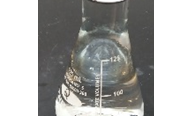Fill the 125-mL Erlenmeyer flask full to the rim with water and measure the volume of the water.  You need to determine the volume of the expanded vapor in the flask, not just where the volume markings are on the flask. Volume of the Erlenmeyer flask =     _______________________ mL

Section 4. Calculations

 Trial 1 Trial 2 Convert your recorded barometric pressure (c) to atmospheres (atm). Assume that the pressure of the gas, P, is the same as the atmospheric pressure when the flask is full of the vapor of the unknown liquid.    Show your equation here: P = ____________ atm P = ____________ atm Convert the volume of the Erlenmeyer flask (e) to liters (l). Assume that the volume of the gas, V, is equal to the volume of the flask.    Show your equation here: V = ___________ l V = ____________ l Convert the temperature of water bath (b) to Kelvin. Assume that the temperature of the gas, T, is the same as the temperature of the water bath when the liquid has completely vaporized.     Show your equation here: T = ___________ K T = ____________ K Using the ideal gas law, PV = nRT, calculate the number of moles, n, of the gas.    Show your equation here: n = ___________ mol n = ____________ mol Calculate the mass of the sample: (d) – (a) ______________ g _______________ g Using the mass and number of moles of the sample, calculate the molar mass of the liquid unknown.    Show your equation here: ______________ g/mol ______________ g/mol Calculate the average molar mass of the liquid unknown. ______________ g/mol

Post Lab Questions:

1.  Obtain the identity of your unknown liquid sample from your instructor. From the chemical formula of the compound, calculate its molar mass. Compare this value with the average molar mass you obtained from this experiment. Calculate the percent error.

1.  There are some experimental errors or facts that could lead to a higher or lower calculated molar mass compared to the true value. In each case below, determine whether the experimental value would be higher or lower than the true value of the molar mass of the compound analyzed. Explain your answer briefly.

1. You took the flask out of the water bath before the entire sample had vaporized.

1. You took the flask out of the water bath before the entire sample had vaporized, and then put it back in the water bath to finish the vaporization process.

1. The temperature of the vapor inside the flask could be lower than the temperature of the water bath that you recorded.

1. You did not wipe off the traces of water outside the flask before weighing it.

1.  When you calculated the moles of the gas (i.e. vapor inside the flask), you used the ideal gas law. However, the vapor is probably not acting like an ‘ideal gas’ under the conditions of this experiment. Explain why this is so. How would this behavior affect the value of the calculated molar mass? Would it be higher or smaller than the true value?

1.  At the end of the experiment, you weighed your unknown sample in the flask after it has condensed back into a liquid.

1. Why can’t you just measure the mass of the liquid that you originally put into the flask at the beginning of the experiment for use in the calculations?

1. How do we take into account the presence of air in the flask?

Experiment_609_Determining the Molar Mass Using Ideal Gas Law_1_2_0 is shared under a CC BY license and was authored, remixed, and/or curated by LibreTexts.